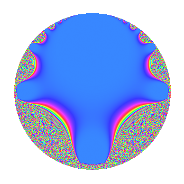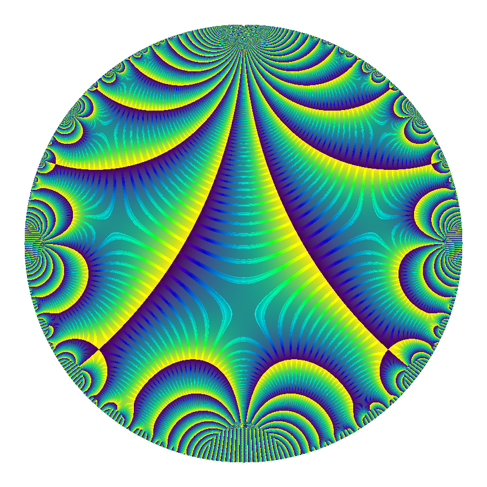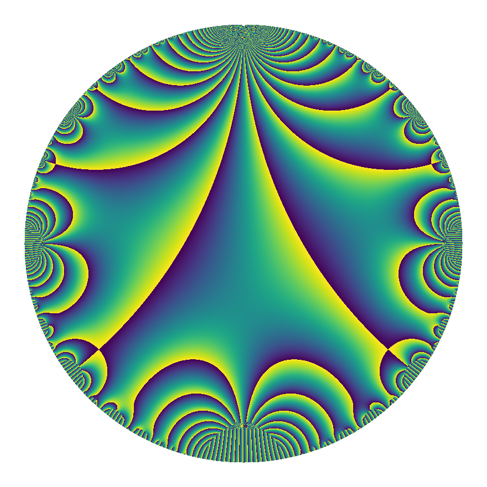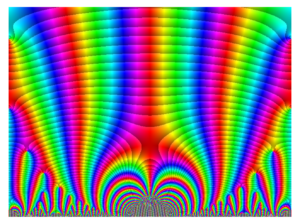## The current cover art for the Proceedings of the Royal Society

The current issue of the Proceedings of the Royal Society A1 features cover artwork made by Vikas Krishnamurthy, Miles Wheeler, Darren Crowdy, Adrian Constantin, and me.A version of the cover pre-addition is the following.This is based on the work in A transformation between stationary point vortex equilibria, which concerns solutions to Euler’s equation for inviscid (2D) fluid motion $$\frac{\partial \mathbf{V}}{\partial t} + (\mathbf{V} \cdot \nabla) \mathbf{V} = – \frac{\nabla p}{p_0},$$ where $\nabla = (\partial/\partial x, \partial / \partial y)$ is the 2D gradient operator. There is a notion of vortices for these systems, and the paper examines configurations of point vortices under certain idealized conditions that leads to particularly nice analysis. In the situation studied, one can sometimes begin with one configurations of point vortices and perform a transformation that yields another, bigger and more complicated configuration.

This is the situation depicted on the cover — begin with a simple configuration and iterate the process. The spiral shape was added afterwards and doesn’t describe underlying mathematical phenomena. The different colors of each vortex shows whether that vortex is a sink or a source, essentially.

I was told most of this after the fact by Miles — who researches fluid dynamics, is a friend from grad school, and was my coauthor on a paper about the mean value theorem. I do not typically think about fluid dynamics (and did not write the paper), and it’s a bit funny how I got involved in the production of this cover. But it was fun, and we produced many arresting images. In the future Miles and I intend to revisit these images and better describe how the various aspects of the image describes and reflects the underlying mathematical behavior.

As a fun aside — we didn’t only produce one image. We made many, and we made many configurations.2 In my work on visualizing modular forms, I developed a few techniques for color selection from matplotlib style colormaps, and produced several variants. I’ve collected a few of these below.

## phase_mag_plot: a sage package for plotting complex functions

Inspired by conversations with Elias Wegert and Frank Farris at the Illustrating Mathematics semester program at ICERM last year, I wrote several plotting libraries for complex plotting. I wrote them with the intention of plotting modular forms in a variety of ways, leading to my talk at Bowdoin in November 2019 and my first post on the CS arxiv.1

I’ve gotten several requests to make these plotting libraries available, and so I’ve made davidlowryduda/phase_mag_plot available on github as a sage library. See the github page and README for examples and up-to-date information.

This version is capable of producing contour-type plots of complex functions.

This does not include any colormap capability yet, as that is a substantially more involved2 process. But at some point in the future, I intend to look at revisiting the complex plotting within sage itself, perhaps updating it to allow plots of this nature.

## Notes from a talk at Dartmouth on the Fibonacci zeta function

I recently gave a talk “at Dartmouth”1. The focus of the talk was the (odd-indexed) Fibonacci zeta function:
$$\sum_{n \geq 1} \frac{1}{F(2n-1)^s},$$
where $F(n)$ is the nth Fibonacci number. The theme is that the Fibonacci zeta function can be recognized as coming from an inner product of automorphic forms, and the continuation of the zeta function can be understood in terms of the spectral expansion of the associated automorphic forms.

This is a talk from ongoing research. I do not yet understand “what’s really going on”. But within the talk I describe a few different generalizations; firstly, there is a generalization to other zeta functions that can be viewed as traces of units on quadratic number fields, and secondly there is a generalization to quadratic forms recognizing solutions to Pell’s equation.

I intend to describe additional ideas from this talk in the coming months, as I figure out how pieces fit together. But for now, here are the slides.

## Pictures of equidistribution – the line

In my previous note, we considered equidistribution of rational points on the circle $X^2 + Y^2 = 2$. This is but one of a large family of equidistribution results that I’m not particularly familiar with.

This note is the first in a series of notes dedicated to exploring this type of equidistribution visually. In this note, we will investigate a simpler case — rational points on the line.

## Points on X^2 + Y^2 = 2 equidistribute with respect to height

When you order rational points on the circle $X^2 + Y^2 = 2$ by height, these points equidistribute.

Stated differently, suppose that $I$ is an arc on the circle $X^2 + Y^2 = 2$. Then asymptotically, the number of rational points on the arc $I$ with height bounded by a number $H$ is equal to what you would expect if $\lvert I\rvert /2\sqrt{2}\pi$ of all points with height up to $H$ were on this arc. Here, $\lvert I\rvert /2\sqrt{2}\pi$ the ratio of the arclength of the arc $I$ with the total circumference of the circle.

This only makes sense if we define the height of a rational point on the circle. Given a point $(a/c, b/c)$ (written in least terms) on the circle, we define the height of this point to be $c$.

In forthcoming work with my frequent collaborators Chan Ieong Kuan, Thomas Hulse, and Alexander Walker, we count three term arithmetic progressions of squares. If $C^2 – B^2 = B^2 – A^2$, then clearly $A^2 + C^2 = 2B^2$, and thus a 3AP of squares corresponds to a rational point on the circle $X^2 + Y^2 = 2$. We compare one of our results to what you would expect from equidistribution. From general principles, we expected such equidistribution to be true. But I wasn’t sure how to prove it.

With helpful assistance from Noam Elkies, Emmanuel Peyre, and John Voight (who each immediately knew how to prove this), I learned how to prove this fact.

The rest of this note contains this proof.

Posted in Expository, Math.NT, Mathematics, sage, sagemath | | 1 Comment

## Proposal for new images for modular forms on the LMFDB

I recently gave a talk about different visualizations of modular forms, including many new visualizations that I have been developing and making. I have continued to develop these images, and I now have a proposal for new visualizations for modular forms in the LMFDB.

To see a current visualization, look at this modular form page. The image from that page (as it is currently) looks like this.This is a plot on a disk model. To make sense of this plot, I note that the real axis in the upper-half-plane model is the circumference of the circle, and the imaginary axis in the upper-half-plane model is the vertical diameter of the circle. In particular, $z = 0$ is the bottom of the circle, $z = i$ is the center of the circle, and $z = \infty$ is the top of the circle. The magnitude is currently displayed — the big blue region is where the magnitude is very small. In a neighborhood of the blue blob, there are a few bands of color that are meaningful — but then things change too quickly and the graph becomes a graph of noise.

I propose one of the following alternatives. I maintain the same badge and model for the space, but I change what is plotted and what colors to use. Also, I plot them larger so that we can get a good look at them; for the LMFDB they would probably be produced at the same (small) size.

## Plots with “Contours”

I have made three plots with contours. They are all morally the same, except for the underlying colorscheme. The “default” sage colorscheme leads to the following plot.The good thing is that it’s visually striking. But I recently learned that this colorscheme is hated, and it’s widely thought to be a poor choice in almost every situation.

A little bit ago, matplotlib added two colorschemes designed to fix the problems with the default colorscheme. (sage’s preferences are behind — the new matplotlib default has changed). This is one of them, called twilight.

##And this is the other default, called viridis. I don’t actually think this should be used, since the hues change from bright yellow to dark blue at complex-argument pi to negative pi. This gives the strong lines, which correspond to those places where the argument of the modular form is pi.Plots without Contours

I’ve also prepared these plots without the contours, and I think they’re quite nice as well.

First jet.Then twilight. At the talk I recently gave, this was the favorite — but I hadn’t yet implemented the contour-plots above for non-default colorschemes.Then viridis. (I’m still not serious about this one — but I think it’s pretty).Note on other Possibilities

There are other possibilities, such as perhaps plotting on a portion of the upper half-plane instead of a disk-model. I describe a few of these possibilities and give examples in the notes from my last talk. I should note that I can now produce contour-type plots there as well, though I haven’t done that.

For fun, here is the default colorscheme, but rotated. This came about accidentally (as did so many other plots in this excursion), but I think it highlights how odd jet is.

## Gathering Opinions

This concludes my proposal. I am collecting opinions. If you are struck by an idea or an opinion and would like to share it with me, please let me know, email me, or leave a comment below.

## Notes behind a talk: visualizing modular forms

Today, I’ll be at Bowdoin College giving a talk on visualizing modular forms. This is a talk about the actual process and choices involved in illustrating a modular form; it’s not about what little lies one might hold in their head in order to form some mental image of a modular form.1This is a talk heavily inspired by the ICERM semester program on Illustrating Mathematics (currently wrapping up). In particular, I draw on2 conversations with Frank Farris (about using color to highlight desired features), Elias Wegert (about using logarithmically scaling contours), Ed Harriss (about the choice of colorscheme), and Brendan Hassett (about overall design choices).

There are very many pictures in the talk!

I wrote a few different complex-plotting routines for this project. At their core, they are based on sage’s complex_plot. There are two major variants that I use.

The first (currently called “ccomplex_plot”. Not a good name) overwrites how sage handles lightness in complex_plot in order to produce “contours” at spots where the magnitude is a two-power. These contours are actually a sudden jump in brightness.

The second (currently called “raw_complex_plot”, also not a good name) is even less formal. It vectorizes the computation and produces an object containing the magnitude and argument information for each pixel to be drawn. It then uses numpy and matplotlib to convert these magnitudes and phases into RGB colors according to a matplotlib-compatible colormap.

I am happy to send either of these pieces of code to anyone who wants to see them, but they are very much written for my own use at the moment. I intend to improve them for general use later, after I’ve experimented further.

In addition, I generated all the images for this talk in a single sagemath jupyter notebook (with the two .spyx cython dependencies I allude to above). This is also available here. (Note that using a service like nbviewer or nbconvert to view or convert it to html might be a reasonable idea).

As a final note, I’ll add that I mistyped several times in the preparation of the images for this talk. Included below are a few of the interesting-looking mistakes. The first two resulted from incorrectly applied conformal mappings, while the third came from incorrectly applied color correction.

Posted in Expository, Math.NT, Mathematics, sage, sagemath, sagemath | | 2 Comments

# Making plots of modular forms¶

Inspired by the images and ideas of Elias Wegert, I thought it might be interesting to attempt to implement a version of his colorizing technique for complex functions in sage. The purpose is ultimately to revisit how one plots modular forms in the LMFDB (see lmfdb.org and click around to see various plots — some are good, others are less good).

The challenge is that plotting a function from $\mathbb{C} \longrightarrow \mathbb{C}$ is that the graph is naturally 4-dimensional, and we are very bad at visualizing 4d things. In fact, we want to use only 2d to visualize it.

A complex number $z = re^{i \theta}$ is determined by the magnitude ($r$) and the argument ($\theta$). Thus
one typical approach to represent the value taken by a function $f$ at a point $z$ is to represent the magnitude of $f(z)$ in terms of the brightness, and to represent the argument in terms of color.

For example, the typical complex space would then look like the following.

## Non-real poles and irregularity of distribution I

$\DeclareMathOperator{\SL}{SL}$ $\DeclareMathOperator{\MT}{MT}$After the positive feedback from the Maine-Quebec Number Theory conference, I have taken some time to write (and slightly strengthen) these results.

We study the general theory of Dirichlet series $D(s) = \sum_{n \geq 1} a(n) n^{-s}$ and the associated summatory function of the coefficients, $A(x) = \sum_{n \leq x}’ a(n)$ (where the prime over the summation means the last term is to be multiplied by $1/2$ if $x$ is an integer). For convenience, we will suppose that the coefficients $a(n)$ are real, that not all $a(n)$ are zero, that each Dirichlet series converges in some half-plane, and that each Dirichlet series has meromorphic continuation to $\mathbb{C}$. Perron’s formula (or more generally, the forward and inverse Mellin transforms) show that $D(s)$ and $A(x)$ are duals and satisfy \begin{equation}\label{eq:basic_duality} \frac{D(s)}{s} = \int_1^\infty \frac{A(x)}{x^{s+1}} dx, \quad A(x) = \frac{1}{2 \pi i} \int_{\sigma – i \infty}^{\sigma + i \infty} \frac{D(s)}{s} x^s ds \end{equation} for an appropriate choice of $\sigma$.

Many results in analytic number theory take the form of showing that $A(x) = \MT(x) + E(x)$ for a “Main Term” $\MT(x)$ and an “Error Term” $E(x)$. Roughly speaking, the terms in the main term $\MT(x)$ correspond to poles from $D(s)$, while $E(x)$ is hard to understand. Upper bounds for the error term give bounds for how much $A(x)$ can deviate from the expected size, and thus describe the regularity in the distribution of the coefficients ${a(n)}$. In this article, we investigate lower bounds for the error term, corresponding to irregularity in the distribution of the coefficients.

To get the best understanding of the error terms, it is often necessary to work with smoothed sums $A_v(x) = \sum_{n \geq 1} a(n) v(n/x)$ for a weight function $v(\cdot)$. In this article, we consider nice weight functions, i.e.\ weight functions with good behavior and whose Mellin transforms have good behavior. For almost all applications, it suffices to consider weight function $v(x)$ that are piecewise smooth on the positive real numbers, and which take values halfway between jump discontinuities.

For a weight function $v(\cdot)$, denote its Mellin transform by \begin{equation} V(s) = \int_0^\infty v(x)x^{s} \frac{dx}{x}. \end{equation} Then we can study the more general dual family \begin{equation}\label{eq:general_duality} D(s) V(s) = \int_1^\infty \frac{A_v(x)}{x^{s+1}} dx, \quad A_v(x) = \frac{1}{2 \pi i} \int_{\sigma – i \infty}^{\sigma + i \infty} D(s) V(s) x^s ds. \end{equation}

We prove two results governing the irregularity of distribution of weighted sums. Firstly, we prove that a non-real pole of $D(s)V(s)$ guarantees an oscillatory error term for $A_v(x)$.

### Theorem 1

Suppose $D(s)V(s)$ has a pole at $s = \sigma_0 + it_0$ with $t_0 \neq 0$ of order $r$. Let $\MT(x)$ be the sum of the residues of $D(s)V(s)X^s$ at all real poles $s = \sigma$ with $\sigma \geq \sigma_0$.Then \begin{equation} \sum_{n \geq 1} a(n) v(\tfrac{n}{x}) – \MT(x) = \Omega_\pm\big( x^{\sigma_0} \log^{r-1} x\big). \end{equation}

Here and below, we use the notation $f(x) = \Omega_+ g(x)$ to mean that there is a constant $k > 0$ such that $\limsup f(x)/\lvert g(x) \rvert > k$ and $f(x) = \Omega_- g(x)$ to mean that $\liminf f(x)/\lvert g(x) \rvert < -k$. When both are true, we write $f(x) = \Omega_\pm g(x)$. This means that $f(x)$ is at least as positive as $\lvert g(x) \rvert$ and at least as negative as $-\lvert g(x) \rvert$ infinitely often.

### Theorem 2

Suppose $D(s)V(s)$ has at least one non-real pole, and that the supremum of the real parts of the non-real poles of $D(s)V(s)$ is $\sigma_0$. Let $\MT(x)$ be the sum of the residues of $D(s)V(s)X^s$ at all real poles $s = \sigma$ with $\sigma \geq \sigma_0$.Then for any $\epsilon > 0$, \begin{equation} \sum_{n \geq 1} a(n) v(\tfrac{n}{x}) – \MT(x) = \Omega_\pm( x^{\sigma_0 – \epsilon} ). \end{equation}

The idea at the core of these theorems is old, and was first noticed during the investigation of the error term in the prime number theorem. To prove them, we generalize proofs given in Chapter 5 of Ingham’s Distribution of Prime Numbers (originally published in 1932, but recently republished). There, Ingham proves that $\psi(x) – x = \Omega_\pm(x^{\Theta – \epsilon})$ and $\psi(x) – x = \Omega_\pm(x^{1/2})$, where $\psi(x) = \sum_{p^n \leq x} \log p$ is Chebyshev’s second function and $\Theta \geq \frac{1}{2}$ is the supremum of the real parts of the non-trivial zeros of $\zeta(s)$. (Peter Humphries let me know that chapter 15 of Montgomery and Vaughan’s text also has these. This text might be more readily available and perhaps in more modern notation. In fact, I have a copy — but I suppose I either never got to chapter 15 or didn’t have it nicely digested when I needed it).

## Motivation and Application

Infinite lines of poorly understood poles appear regularly while studying shifted convolution series of the shape \begin{equation} D(s) = \sum_{n \geq 1} \frac{a(n) a(n \pm h)}{n^s} \end{equation} for a fixed $h$. When $a(n)$ denotes the (non-normalized) coefficients of a weight $k$ cuspidal Hecke eigenform on a congruence subgroup of $\SL(2, \mathbb{Z})$, for instance, meromorphic continuation can be gotten for the shifted convolution series $D(s)$ through spectral expansion in terms of Maass forms and Eisenstein series, and the Maass forms contribute infinite lines of poles.

Explicit asymptotics take the form \begin{equation} \sum_{n \geq 1} a(n)a(n-h) e^{-n/X} = \sum_j C_j X^{\frac{1}{2} + \sigma_j + it_j} \log^m X \end{equation} where neither the residues nor the imaginary parts $it_j$ are well-understood. Might it be possible for these infinitely many rapidly oscillating terms to experience massive cancellation for all $X$? The theorems above prove that this is not possible.

In this case, applying Theorem 2 with the Perron-weight \begin{equation} v(x) = \begin{cases} 1 & x < 1 \\ \frac{1}{2} & x = 1 \\ 0 & x > 1 \end{cases} \end{equation} shows that \begin{equation} \sideset{}{‘}\sum_{n \leq X} \frac{a(n)a(n-h)}{n^{k-1}} = \Omega_\pm(\sqrt X). \end{equation} Similarly, Theorem 1 shows that \begin{equation} \sideset{}{‘}\sum_{n \leq X} \frac{a(n)a(n-h)}{n^{k-1}} = \Omega_\pm(X^{\frac{1}{2} + \Theta – \epsilon}), \end{equation} where $\Theta < 7/64$ is the supremum of the deviations to Selberg’s Eigenvalue Conjecture (sometimes called the the non-arithmetic Ramanujan Conjecture).

More generally, these shifted convolution series appear when studying the sizes of sums of coefficients of modular forms. A few years ago, Hulse, Kuan, Walker, and I began an investigation of the Dirichlet series whose coefficients were themselves $\lvert A(n) \rvert^2$ (where $A(n)$ is the sum of the first $n$ coefficients of a modular form) was shown to have meromorphic continuation to $\mathbb{C}$. The behavior of the infinite lines of poles in the discrete spectrum played an important role in the analysis, but we did not yet understand how they affected the resulting asymptotics. I plan on revisiting these results, and others, with these results in mind.

## Proofs

The proofs of these results will soon appear on the arXiv.

## Notes from a talk at the Maine-Quebec Number Theory Conference

Today I will be giving a talk at the Maine-Quebec Number Theory conference. Each year that I attend this conference, I marvel at how friendly and inviting an environment it is — I highly recommend checking the conference out (and perhaps modelling other conferences after it).

The theme of my talk is about spectral poles and their contribution towards asymptotics (especially of error terms). I describe a few problems in which spectral poles appear in asymptotics. Unlike the nice simple cases where a single pole (or possibly a few poles) appear, in these cases infinite lines of poles appear.

For a bit over a year, I have encountered these and not known what to make of them. Could you have the pathological case that residues of these poles generically cancel? Could they combine to be larger than expected? How do we make sense of them?

The resolution came only very recently.1

I will later write a dedicated note to this new idea (involving Dirichlet integrals and Landau’s theorem in this context), but for now — here are the slides for my talk.

Posted in Expository, Math.NT, Mathematics | | 2 Comments